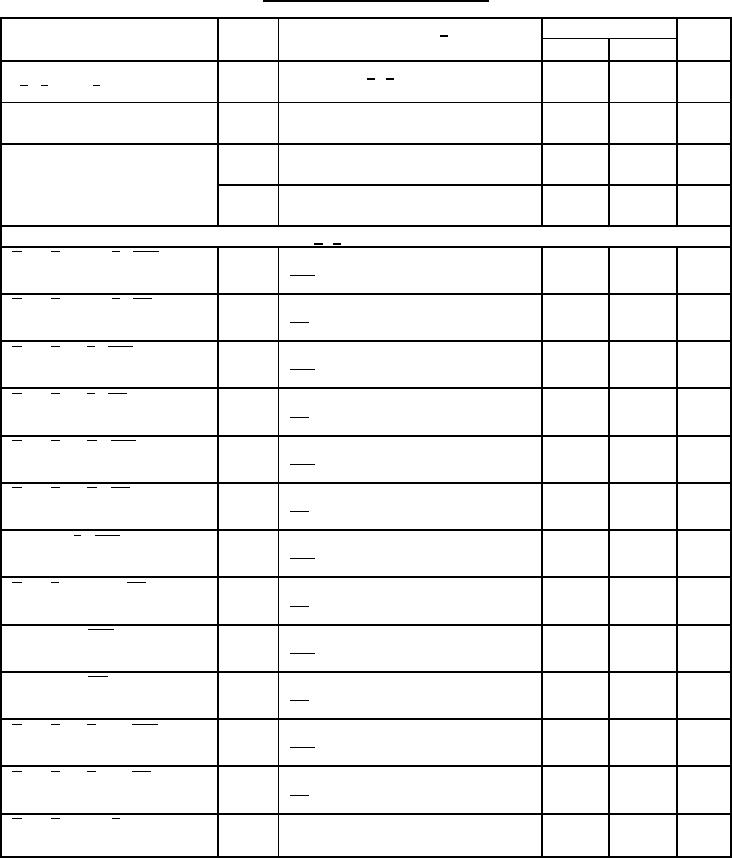Click here to make tpub.com your Home Page Page Title: Table 1. Electrical performance characteristics-cont. Back | Up | NextWeb www.tpub.comHome

Information Categories
Aerographer
Automotive
Aviation
Construction
Diving
Draftsman
Engineering
Electronics
Food and Cooking
Logistics
Math
Medical
Music
Nuclear Fundamentals
Photography
ReligionMIL-M-38510/308B
TABLE I. Electrical performance characteristics - Continued.
Conditions 1/
Limits
Unit
Test
Symbol
-55C TC +125C
Min
Max
Short circuit output current at
IOS3
VCC = 5.5 V, 2/ 4/
-15
-100
mA
P , F 0 thru F 3 outputs
A
Collector cutoff current at
ICEX
VCC = 4.5 V, V(A = B) = 5.5 V,
100
A = B output
all input = 4.5 V
Supply current
ICC1
VCC = 5.5 V,
35
mA
S0 thru S3, M, and A = 5.5 V
ICC2
VCC = 5.5 V, S0 thru S3 and M = 5.5 V,
35
mA
all others grounded
Propagation delay times, high to low level output: 5/, 6/
VCC = 5.0 V, See figure 4
2
37
ns
tPHL1
A i or B i to any F , sum mode
sum mode test table
VCC = 5.0 V, See figure 4
2
55
ns
tPHL2
A i or B i to any F , diff mode
diff mode test table
VCC = 5.0 V, See figure 4
2
52
ns
tPHL3
A i or B i to P , sum mode
sum mode test table
VCC = 5.0 V, See figure 4
2
57
ns
tPHL4
A i or B i to P , diff mode
diff mode test table
VCC = 5.0 V, See figure 4
2
42
ns
tPHL5
A i or B i to G , sum mode
sum mode test table
VCC = 5.0 V, See figure 4
2
55
ns
tPHL6
A i or B i to G , diff mode
diff mode test table
VCC = 5.0 V, See figure 4
2
37
ns
tPHL7
Cn to any F , sum mode
sum mode test table
VCC = 5.0 V, See figure 4
2
100
ns
tPHL8
A i to B i to A = B, diff mode
diff mode test table
VCC = 5.0 V, See figure 4
2
37
ns
tPHL9
Cn to Cn+4, sum mode
sum mode test table
VCC = 5.0 V, See figure 4
2
37
ns
tPHL10
Cn to Cn+4, diff mode
diff mode test table
VCC = 5.0 V, See figure 4
2
64
ns
tPHL11
A i or B i to C n+4, sum mode
sum mode test table
VCC = 5.0 V, See figure 4
2
60
ns
tPHL12
A i or B i to C n+4, diff mode
diff mode test table
tPHL13
VCC = 5.0 V, See figure 4
2
48
ns
A i or B i to any F , logic mode
logic mode test table
See footnotes at end of table.
5Integrated Publishing, Inc. - A (SDVOSB) Service Disabled Veteran Owned Small Business